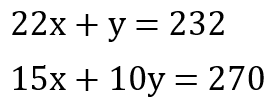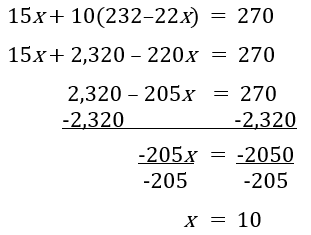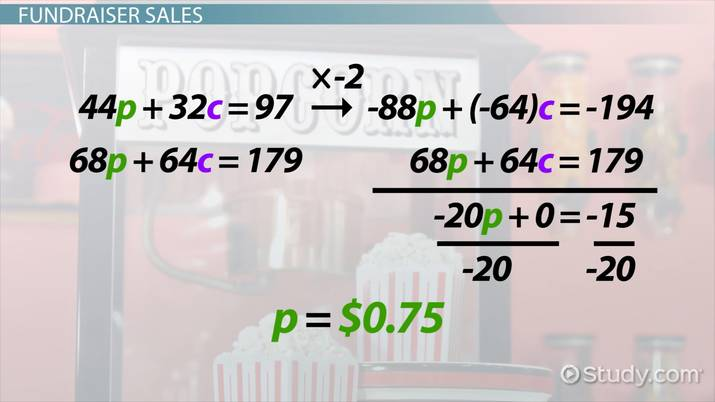System of Equations Word Problem Examples

Lesson Transcript
Instructor: Mia Primas

Mia has taught math and science and has a Master's Degree in Secondary Teaching.

System of equations problems involve finding multiple missing values. Practice solving these multi-equations with systems of equations word problem examples that focus on realistic scenarios, like getting paid and calculating fundraiser sales. Updated: 12/21/2021

Getting Paid

Systems of equations have two or more equations with two or more variables that are solved simultaneously. The goal is to find a value for each variable that satisfies the equations. In the following examples, we'll create systems of equations based on the word problems. We will then use substitution and elimination strategies to solve them.

Example 1

My younger cousins worked part-time jobs over the summer to save money for a car. The job paid different rates for working weekdays and weekends. During the first week, one cousin worked 22 hours during the week and one hour on the weekend, earning \$232. Her sister earned \$270 by working 15 hours during the week and 10 hours on the weekend. What was the hourly pay rate for working weekdays and weekends?

When solving word problems, it's important to understand what you are asked to find. This problem is asking us to find the hourly pay rate for weekdays and the hourly pay rate for weekends. We'll need to use different variables to represent each unknown. Let's use x for the weekday rate and y for the weekend rate. Now we can use the other information from the word problem to create equations that we will use to solve for the variables.

One cousin worked 22 weekday hours and one weekend hour for a total of \$232. We can represent this with the equation 22x + y = 232. Using the pay for the other cousin, we get the equation 15x + 10y = 270. Since there are two equations with the same two variables, we can set them up as a system of equations and solve them simultaneously.The system of equations can be solved using the substitution method, which involves using an expression from one equation to substitute for one of the variables in the other equation. The first equation can be solved for y by subtracting 22x from both sides of the equation. This leaves us with y = 232 - 22x. Since y is equal to the expression 232 - 22x, we can substitute the expression for y in the second equation. Thus, the equation becomes 15x + 10 (232 - 22x) = 270. We now have one equation with one variable to solve, which works out like this:We now know that the hourly pay rate for weekdays is ten dollars. By substituting ten for x in one of the equations, we can solve for y. Using the revised version of the first equation, we get y = 232 - 22 (10) = 12.

Now that the values of both variables are known, the word problem is solved. The hourly pay rate is ten dollars for weekdays and twelve dollars for weekends.An error occurred trying to load this video.

Try refreshing the page, or contact customer support.

Coming up next: Consistent System of Equations: Definition & Examples

You're on a roll. Keep up the good work!

Replay
Your next lesson will play in 10 seconds
• 0:04 Getting Paid
• 3:09 Fundraiser Sales
• 5:50 Lesson Summary
Save Save

Want to watch this again later?

Timeline
Autoplay
Autoplay
Speed Speed

Fundraiser Sales

Okay, let's now go through another example using the elimination method this time.

Example 2

A high school soccer team held a fundraiser to raise money for new uniforms. They sold cookies and flavored popcorn. On the first day, they sold 44 bags of popcorn and 32 boxes of cookies for a total of \$97. On the second day, they raised \$179 by selling 68 bags of popcorn and 64 boxes of cookies. How much did they charge for each cookie and bag of popcorn?

To unlock this lesson you must be a Study.com Member.

Register to view this lesson

Are you a student or a teacher?